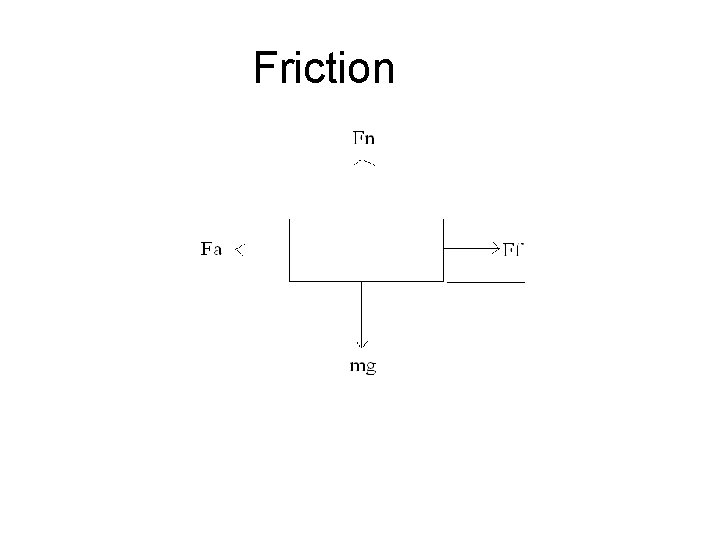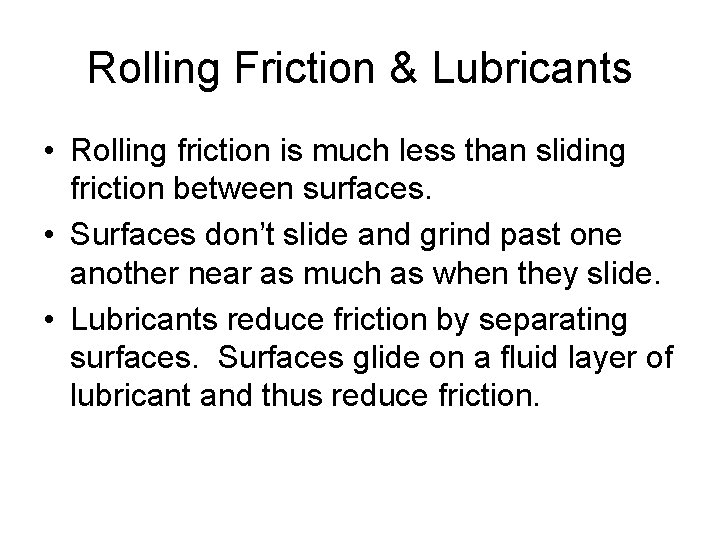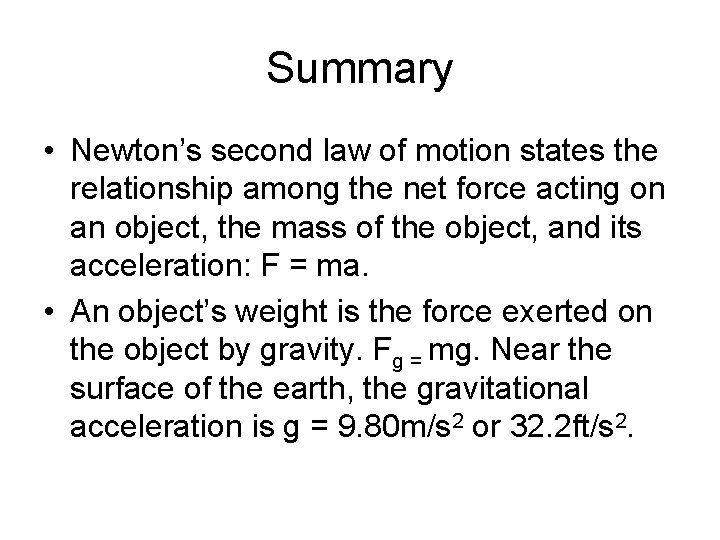# Chapter 4 Resistance 4 1 Resistance in Mechanical

• Slides: 9Chapter 4 - Resistance 4. 1 Resistance in Mechanical SystemsFrictionFriction • • mg = weight Fn = normal force Ff = frictional force Fa = applied forceFriction formula • Ff = m. Fn m = coefficient of friction The normal force is the reaction force of the surface to the weight of the object. It is perpendicular or “normal” to the surface. On a flat and level surface, the normal force is equal to the weight of the object.Friction may be static (starting) or kinetic (moving). Thus there is a coefficient of static friction ( ms) and a coefficient of kinetic friction ( mk) Kinetic friction is usually less than static friction If an object is pulled at a constant speed across a flat, level surface, the frictional force will equal the force of the pull (the applied force) and the normal force will equal the weight of the object. Thus Ff = m. Fn or Fa = mmg Thus m = Fa / mg i. e. The coefficient of friction is the ratio of how hard you have to push or pull to the weight of the object.Rolling Friction & Lubricants • Rolling friction is much less than sliding friction between surfaces. • Surfaces don’t slide and grind past one another near as much as when they slide. • Lubricants reduce friction by separating surfaces. Surfaces glide on a fluid layer of lubricant and thus reduce friction.Summary • Newton’s second law of motion states the relationship among the net force acting on an object, the mass of the object, and its acceleration: F = ma. • An object’s weight is the force exerted on the object by gravity. Fg = mg. Near the surface of the earth, the gravitational acceleration is g = 9. 80 m/s 2 or 32. 2 ft/s 2.Summary – cont. • Friction causes resistance in mechanical systems. • To start an object moving, you must overcome the force of the static friction. To keep an object moving at a constant speed, you must overcome the force of kinetic friction. • According to the linear model, the force of friction between two surfaces is directly proportional to the normal force pushing the surfaces together.More Summary • Lubrication and rollers reduce friction. Lubricants separate the two sliding surfaces with a layer of fluid.